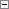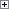Rounds a DateTime.

NewDateTime := ROUNDDATETIME(Datetime [, Precision][, Direction])

Parameters

Datetime

Type: DateTime

The DateTime that you want to round.

Precision

Type: BigInteger

This optional parameter determines the precision used when rounding. The default value is 1000, which rounds to the nearest second.

You can only use positive BigIntegers.

Direction

Type: Text or Code

This optional parameter specifies how to round the DateTime. The default rounding method is '='. You can change the method by using the following options:

• '=' rounds up or down to the nearest value (default). Values of 5 or greater are rounded up. Values less than 5 are rounded down.
• '>' rounds up
• '<' rounds downProperty Value/Return Value

Type: DateTime

The rounded result.Remarks

A variable of type DateTime is stored as a 64 bit integer. The integer value is the number of milliseconds since year 0. When you call ROUNDDATETIME, the DateTime integer value is rounded as a numeric variable.

If you use 1 for the Precision parameter, then the resulting DateTime is rounded to the nearest millisecond. If you use 1000 for the Precision parameter, which is the default value, then the resulting DateTime will be rounded to the nearest second.

We recommend that you do not use a value greater than 60*60*1000, which is the number of milliseconds in an hour, for the Precision parameter. The Regional and Language Options in Windows affect how the hour and date parts of a DateTime are rounded. To display a DateTime in a specific format, we recommend that you use the FORMAT Function (Code, Text) instead of the ROUNDDATETIME function.Example

This example shows how to use the ROUNDDATETIME function to round to the nearest second. This example requires that you create the following text constant in the C/AL Globals window.

Text constant name ENU value

Text000

ROUNDDATETIME(%1, %2) returns %3.Copy Code
DateToRound := 112708D;
TimeToRound := 093524.567T;
DateTimeToRound := CREATEDATETIME(DateToRound, TimeToRound);
Precision := 1000L;
FormatString := '<Month,2>/<Day,2>/<Year> <Hours24,2>:<Minutes,2>:<Seconds,2>.<Thousands,3>';
Result := ROUNDDATETIME(DateTimeToRound, Precision);
MESSAGE(TEXT000, Format(DateTimeToRound,0,FormatString), Precision, Format(Result,0,FormatString));

The message window displays the following:

ROUNDDATETIME(11/27/08 09:35:24.567, 1000) returns 11/27/08 09:35:25.000.